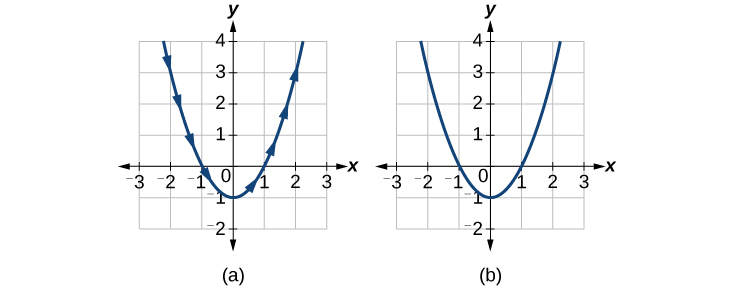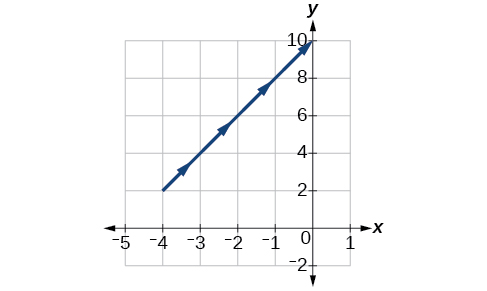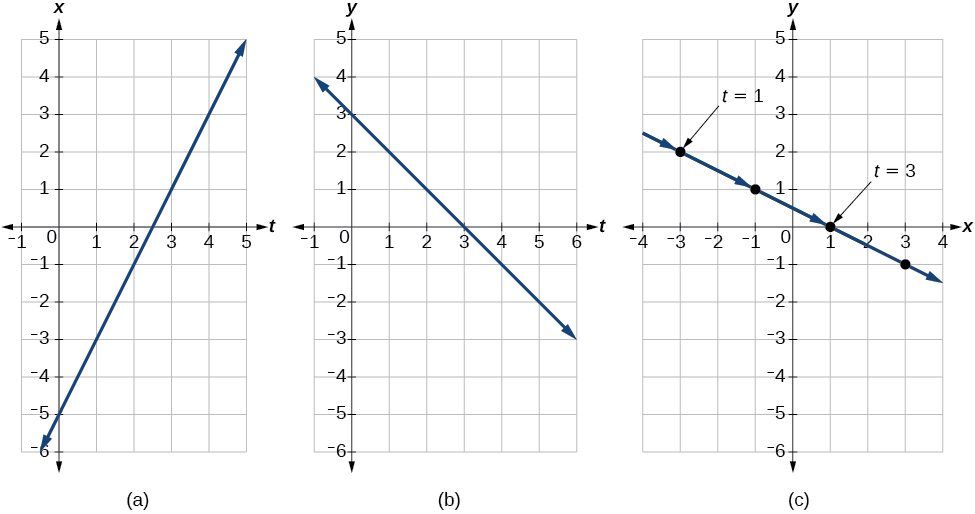# 8.6 Parametric equations  (Page 2/6)

 Page 2 / 6

However, if we were to graph each equation on its own, each one would pass the vertical line test and therefore would represent a function. In some instances, the concept of breaking up the equation for a circle into two functions is similar to the concept of creating parametric equations, as we use two functions to produce a non-function. This will become clearer as we move forward.

## Parametric equations

Suppose $\text{\hspace{0.17em}}t\text{\hspace{0.17em}}$ is a number on an interval, $\text{\hspace{0.17em}}I.\text{\hspace{0.17em}}$ The set of ordered pairs, $\text{\hspace{0.17em}}\left(x\left(t\right),\text{\hspace{0.17em}}\text{\hspace{0.17em}}y\left(t\right)\right),\text{\hspace{0.17em}}$ where $\text{\hspace{0.17em}}x=f\left(t\right)\text{\hspace{0.17em}}$ and $\text{\hspace{0.17em}}y=g\left(t\right),$ forms a plane curve based on the parameter $\text{\hspace{0.17em}}t.\text{\hspace{0.17em}}$ The equations $\text{\hspace{0.17em}}x=f\left(t\right)\text{\hspace{0.17em}}$ and $\text{\hspace{0.17em}}y=g\left(t\right)\text{\hspace{0.17em}}$ are the parametric equations.

## Parameterizing a curve

Parameterize the curve $\text{\hspace{0.17em}}y={x}^{2}-1\text{\hspace{0.17em}}$ letting $\text{\hspace{0.17em}}x\left(t\right)=t.\text{\hspace{0.17em}}$ Graph both equations.

If $\text{\hspace{0.17em}}x\left(t\right)=t,\text{\hspace{0.17em}}$ then to find $\text{\hspace{0.17em}}y\left(t\right)\text{\hspace{0.17em}}$ we replace the variable $\text{\hspace{0.17em}}x\text{\hspace{0.17em}}$ with the expression given in $\text{\hspace{0.17em}}x\left(t\right).\text{\hspace{0.17em}}$ In other words, $\text{\hspace{0.17em}}y\left(t\right)={t}^{2}-1.$ Make a table of values similar to [link] , and sketch the graph.

$t$ $x\left(t\right)$ $y\left(t\right)$
$-4$ $-4$ $y\left(-4\right)={\left(-4\right)}^{2}-1=15$
$-3$ $-3$ $y\left(-3\right)={\left(-3\right)}^{2}-1=8$
$-2$ $-2$ $y\left(-2\right)={\left(-2\right)}^{2}-1=3$
$-1$ $-1$ $y\left(-1\right)={\left(-1\right)}^{2}-1=0$
$0$ $0$ $y\left(0\right)={\left(0\right)}^{2}-1=-1$
$1$ $1$ $y\left(1\right)={\left(1\right)}^{2}-1=0$
$2$ $2$ $y\left(2\right)={\left(2\right)}^{2}-1=3$
$3$ $3$ $y\left(3\right)={\left(3\right)}^{2}-1=8$
$4$ $4$ $y\left(4\right)={\left(4\right)}^{2}-1=15$

See the graphs in [link] . It may be helpful to use the TRACE feature of a graphing calculator to see how the points are generated as $\text{\hspace{0.17em}}t\text{\hspace{0.17em}}$ increases.(a) Parametric   y ( t ) = t 2 − 1   (b) Rectangular   y = x 2 − 1

Construct a table of values and plot the parametric equations: $\text{\hspace{0.17em}}x\left(t\right)=t-3,\text{\hspace{0.17em}}\text{\hspace{0.17em}}y\left(t\right)=2t+4;\text{\hspace{0.17em}}\text{\hspace{0.17em}}\text{\hspace{0.17em}}-1\le t\le 2.$

 $t$ $x\left(t\right)$ $y\left(t\right)$ $-1$ $-4$ $2$ $0$ $-3$ $4$ $1$ $-2$ $6$ $2$ $-1$ $8$## Finding a pair of parametric equations

Find a pair of parametric equations that models the graph of $\text{\hspace{0.17em}}y=1-{x}^{2},\text{\hspace{0.17em}}$ using the parameter $\text{\hspace{0.17em}}x\left(t\right)=t.\text{\hspace{0.17em}}$ Plot some points and sketch the graph.

If $\text{\hspace{0.17em}}x\left(t\right)=t\text{\hspace{0.17em}}$ and we substitute $\text{\hspace{0.17em}}t\text{\hspace{0.17em}}$ for $\text{\hspace{0.17em}}x\text{\hspace{0.17em}}$ into the $\text{\hspace{0.17em}}y\text{\hspace{0.17em}}$ equation, then $\text{\hspace{0.17em}}y\left(t\right)=1-{t}^{2}.\text{\hspace{0.17em}}$ Our pair of parametric equations is

$\begin{array}{l}x\left(t\right)=t\\ y\left(t\right)=1-{t}^{2}\end{array}$

To graph the equations, first we construct a table of values like that in [link] . We can choose values around $\text{\hspace{0.17em}}t=0,\text{\hspace{0.17em}}$ from $\text{\hspace{0.17em}}t=-3\text{\hspace{0.17em}}$ to $\text{\hspace{0.17em}}t=3.\text{\hspace{0.17em}}$ The values in the $\text{\hspace{0.17em}}x\left(t\right)\text{\hspace{0.17em}}$ column will be the same as those in the $\text{\hspace{0.17em}}t\text{\hspace{0.17em}}$ column because $\text{\hspace{0.17em}}x\left(t\right)=t.\text{\hspace{0.17em}}$ Calculate values for the column $\text{\hspace{0.17em}}y\left(t\right).\text{\hspace{0.17em}}$

$t$ $x\left(t\right)=t$ $y\left(t\right)=1-{t}^{2}$
$-3$ $-3$ $y\left(-3\right)=1-{\left(-3\right)}^{2}=-8$
$-2$ $-2$ $y\left(-2\right)=1-{\left(-2\right)}^{2}=-3$
$-1$ $-1$ $y\left(-1\right)=1-{\left(-1\right)}^{2}=0$
$0$ $0$ $y\left(0\right)=1-0=1$
$1$ $1$ $y\left(1\right)=1-{\left(1\right)}^{2}=0$
$2$ $2$ $y\left(2\right)=1-{\left(2\right)}^{2}=-3$
$3$ $3$ $y\left(3\right)=1-{\left(3\right)}^{2}=-8$

The graph of $\text{\hspace{0.17em}}y=1-{t}^{2}\text{\hspace{0.17em}}$ is a parabola facing downward, as shown in [link] . We have mapped the curve over the interval $\text{\hspace{0.17em}}\left[-3,\text{\hspace{0.17em}}3\right],$ shown as a solid line with arrows indicating the orientation of the curve according to $\text{\hspace{0.17em}}t.\text{\hspace{0.17em}}$ Orientation refers to the path traced along the curve in terms of increasing values of $\text{\hspace{0.17em}}t.\text{\hspace{0.17em}}$ As this parabola is symmetric with respect to the line $\text{\hspace{0.17em}}x=0,\text{\hspace{0.17em}}$ the values of $\text{\hspace{0.17em}}x\text{\hspace{0.17em}}$ are reflected across the y -axis.

Parameterize the curve given by $\text{\hspace{0.17em}}x={y}^{3}-2y.$

$\begin{array}{l}x\left(t\right)={t}^{3}-2t\\ y\left(t\right)=t\end{array}$

## Finding parametric equations that model given criteria

An object travels at a steady rate along a straight path $\text{\hspace{0.17em}}\left(-5,\text{\hspace{0.17em}}3\right)\text{\hspace{0.17em}}$ to $\text{\hspace{0.17em}}\left(3,\text{\hspace{0.17em}}-1\right)\text{\hspace{0.17em}}$ in the same plane in four seconds. The coordinates are measured in meters. Find parametric equations for the position of the object.

The parametric equations are simple linear expressions, but we need to view this problem in a step-by-step fashion. The x -value of the object starts at $\text{\hspace{0.17em}}-5\text{\hspace{0.17em}}$ meters and goes to 3 meters. This means the distance x has changed by 8 meters in 4 seconds, which is a rate of or $\text{\hspace{0.17em}}2\text{\hspace{0.17em}}\text{m}/\text{s}.\text{\hspace{0.17em}}$ We can write the x -coordinate as a linear function with respect to time as $\text{\hspace{0.17em}}x\left(t\right)=2t-5.\text{\hspace{0.17em}}$ In the linear function template $\text{\hspace{0.17em}}y=mx+b,2t=mx\text{\hspace{0.17em}}$ and $\text{\hspace{0.17em}}-5=b.$

Similarly, the y -value of the object starts at 3 and goes to $\text{\hspace{0.17em}}-1,\text{\hspace{0.17em}}$ which is a change in the distance y of −4 meters in 4 seconds, which is a rate of or $\text{\hspace{0.17em}}-1\text{m}/\text{s}.\text{\hspace{0.17em}}$ We can also write the y -coordinate as the linear function $\text{\hspace{0.17em}}y\left(t\right)=-t+3.\text{\hspace{0.17em}}$ Together, these are the parametric equations for the position of the object, where $\text{\hspace{0.17em}}x\text{\hspace{0.17em}}$ and $\text{\hspace{0.17em}}y\text{\hspace{0.17em}}$ are expressed in meters and $\text{\hspace{0.17em}}t\text{\hspace{0.17em}}$ represents time:

$\begin{array}{l}x\left(t\right)=2t-5\hfill \\ y\left(t\right)=-t+3\hfill \end{array}$

Using these equations, we can build a table of values for $\text{\hspace{0.17em}}t,x,\text{\hspace{0.17em}}$ and $\text{\hspace{0.17em}}y$ (see [link] ). In this example, we limited values of $\text{\hspace{0.17em}}t\text{\hspace{0.17em}}$ to non-negative numbers. In general, any value of $\text{\hspace{0.17em}}t\text{\hspace{0.17em}}$ can be used.

$t$ $x\left(t\right)=2t-5$ $y\left(t\right)=-t+3$
$0$ $x=2\left(0\right)-5=-5$ $y=-\left(0\right)+3=3$
$1$ $x=2\left(1\right)-5=-3$ $y=-\left(1\right)+3=2$
$2$ $x=2\left(2\right)-5=-1$ $y=-\left(2\right)+3=1$
$3$ $x=2\left(3\right)-5=1$ $y=-\left(3\right)+3=0$
$4$ $x=2\left(4\right)-5=3$ $y=-\left(4\right)+3=-1$

From this table, we can create three graphs, as shown in [link] .(a) A graph of   x   vs.   t ,   representing the horizontal position over time. (b) A graph of y vs.   t ,   representing the vertical position over time. (c) A graph of   y   vs.   x ,   representing the position of the object in the plane at time   t .

how fast can i understand functions without much difficulty
what is set?
a colony of bacteria is growing exponentially doubling in size every 100 minutes. how much minutes will it take for the colony of bacteria to triple in size
I got 300 minutes. is it right?
Patience
no. should be about 150 minutes.
Jason
It should be 158.5 minutes.
Mr
ok, thanks
Patience
100•3=300 300=50•2^x 6=2^x x=log_2(6) =2.5849625 so, 300=50•2^2.5849625 and, so, the # of bacteria will double every (100•2.5849625) = 258.49625 minutes
Thomas
what is the importance knowing the graph of circular functions?
can get some help basic precalculus
What do you need help with?
Andrew
how to convert general to standard form with not perfect trinomial
can get some help inverse function
ismail
Rectangle coordinate
how to find for x
it depends on the equation
Robert
yeah, it does. why do we attempt to gain all of them one side or the other?
Melissa
whats a domain
The domain of a function is the set of all input on which the function is defined. For example all real numbers are the Domain of any Polynomial function.
Spiro
Spiro; thanks for putting it out there like that, 😁
Melissa
foci (–7,–17) and (–7,17), the absolute value of the differenceof the distances of any point from the foci is 24.
difference between calculus and pre calculus?
give me an example of a problem so that I can practice answering
x³+y³+z³=42
Robert
dont forget the cube in each variable ;)
Robert
of she solves that, well ... then she has a lot of computational force under her command ....
Walter
what is a function?
I want to learn about the law of exponent
explain this

#### Get Jobilize Job Search Mobile App in your pocket Now!ByByBy Brooke DelaneyBy OpenStaxBy Rohini AjayBy JavaChamp TeamBy OpenStaxBy OpenStaxByBy OpenStaxBy Madison ChristianBy OpenStax